# Understanding Convolutions

In a previous post, we built up an understanding of convolutional neural networks, without referring to any significant mathematics. To go further, however, we need to understand convolutions.

If we just wanted to understand convolutional neural networks, it might suffice to roughly understand convolutions. But the aim of this series is to bring us to the frontier of convolutional neural networks and explore new options. To do that, we’re going to need to understand convolutions very deeply.

Thankfully, with a few examples, convolution becomes quite a straightforward idea.

## Lessons from a Dropped Ball

Imagine we drop a ball from some height onto the ground, where it only has one dimension of motion. How likely is it that a ball will go a distance cc if you drop it and then drop it again from above the point at which it landed?

Let’s break this down. After the first drop, it will land aa units away from the starting point with probability f(a)f(a), where ff is the probability distribution.

Now after this first drop, we pick the ball up and drop it from another height above the point where it first landed. The probability of the ball rolling bb units away from the new starting point is g(b)g(b), where gg may be a different probability distribution if it’s dropped from a different height.If we fix the result of the first drop so we know the ball went distance aa, for the ball to go a total distance cc, the distance traveled in the second drop is also fixed at bb, where a+b=ca+b=c. So the probability of this happening is simply f(a)g(b)f(a)⋅g(b).1

Let’s think about this with a specific discrete example. We want the total distance cc to be 3. If the first time it rolls, a=2a=2, the second time it must roll b=1b=1 in order to reach our total distance a+b=3a+b=3. The probability of this is f(2)g(1)f(2)⋅g(1).However, this isn’t the only way we could get to a total distance of 3. The ball could roll 1 units the first time, and 2 the second. Or 0 units the first time and all 3 the second. It could go any aaand bb, as long as they add to 3.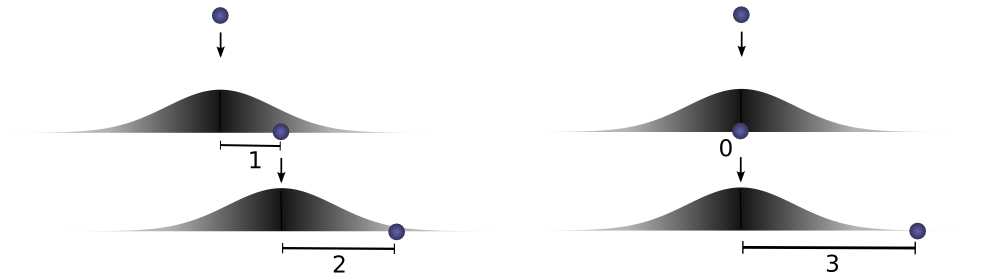The probabilities are f(1)g(2)f(1)⋅g(2) and f(0)g(3)f(0)⋅g(3), respectively.

In order to find the total likelihood of the ball reaching a total distance of cc, we can’t consider only one possible way of reaching cc. Instead, we consider all the possible ways of partitioning ccinto two drops aa and bb and sum over the probability of each way.

...  f(0)g(3) + f(1)g(2) + f(2)g(1)  ...…  f(0)⋅g(3) + f(1)⋅g(2) + f(2)⋅g(1)  …

We already know that the probability for each case of a+b=ca+b=c is simply f(a)g(b)f(a)⋅g(b). So, summing over every solution to a+b=ca+b=c, we can denote the total likelihood as:

a+b=cf(a)g(b)∑a+b=cf(a)⋅g(b)

Turns out, we’re doing a convolution! In particular, the convolution of ff and gg, evluated at cc is defined:

(fg)(c)=a+b=cf(a)g(b)    (f∗g)(c)=∑a+b=cf(a)⋅g(b)

If we substitute b=cab=c−a, we get:

(fg)(c)=af(a)g(ca)(f∗g)(c)=∑af(a)⋅g(c−a)

This is the standard definition2 of convolution.

To make this a bit more concrete, we can think about this in terms of positions the ball might land. After the first drop, it will land at an intermediate position aa with probability f(a)f(a). If it lands at aa, it has probability g(ca)g(c−a) of landing at a position cc.To get the convolution, we consider all intermediate positions.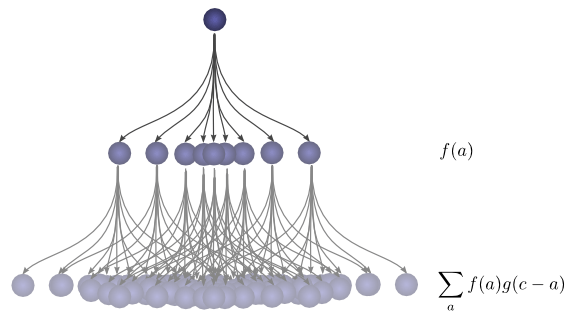## Visualizing Convolutions

There’s a very nice trick that helps one think about convolutions more easily.

First, an observation. Suppose the probability that a ball lands a certain distance xx from where it started is f(x)f(x). Then, afterwards, the probability that it started a distance xx from where it landed is f(x)f(−x).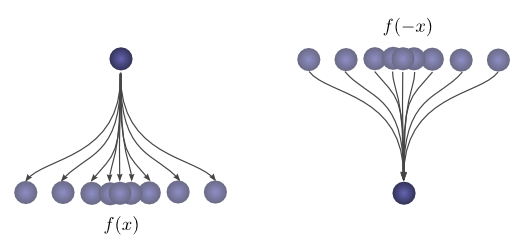If we know the ball lands at a position cc after the second drop, what is the probability that the previous position was aa?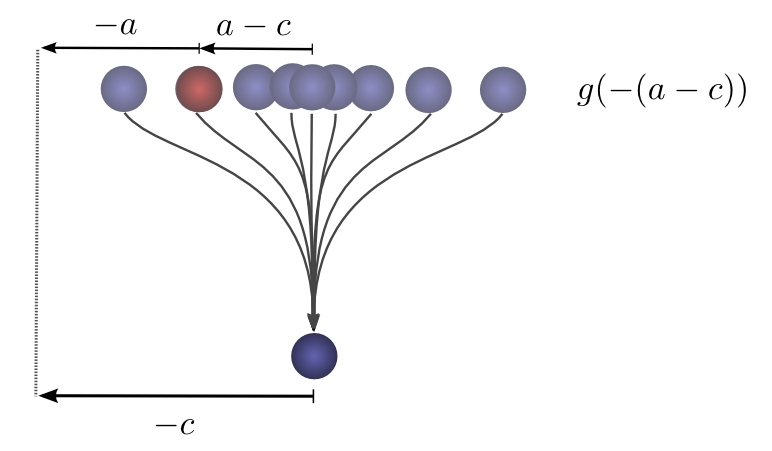So the probability that the previous position was aa is g((ac))=g(ca)g(−(a−c))=g(c−a).

Now, consider the probability each intermediate position contributes to the ball finally landing at cc. We know the probability of the first drop putting the ball into the intermediate position a is f(a)f(a). We also know that the probability of it having been in aa, if it lands at cc is g(ca)g(c−a).Summing over the aas, we get the convolution.

The advantage of this approach is that it allows us to visualize the evaluation of a convolution at a value cc in a single picture. By shifting the bottom half around, we can evaluate the convolution at other values of cc. This allows us to understand the convolution as a whole.

For example, we can see that it peaks when the distributions align.And shrinks as the intersection between the distributions gets smaller.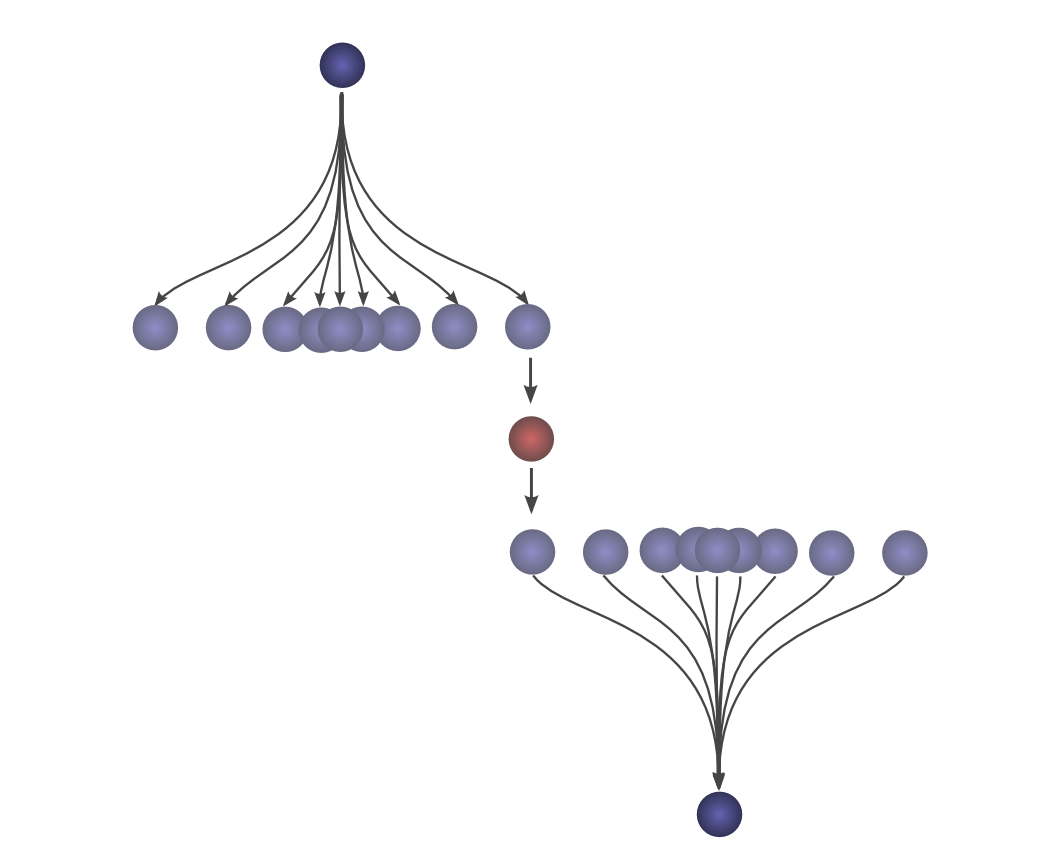By using this trick in an animation, it really becomes possible to visually understand convolutions.

Below, we’re able to visualize the convolution of two box functions: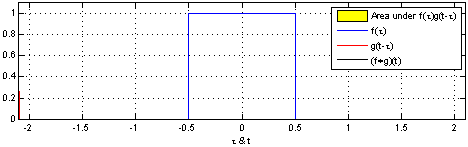From Wikipedia

Armed with this perspective, a lot of things become more intuitive.

Let’s consider a non-probabilistic example. Convolutions are sometimes used in audio manipulation. For example, one might use a function with two spikes in it, but zero everywhere else, to create an echo. As our double-spiked function slides, one spike hits a point in time first, adding that signal to the output sound, and later, another spike follows, adding a second, delayed copy.

## Higher Dimensional Convolutions

Convolutions are an extremely general idea. We can also use them in a higher number of dimensions.

Let’s consider our example of a falling ball again. Now, as it falls, it’s position shifts not only in one dimension, but in two.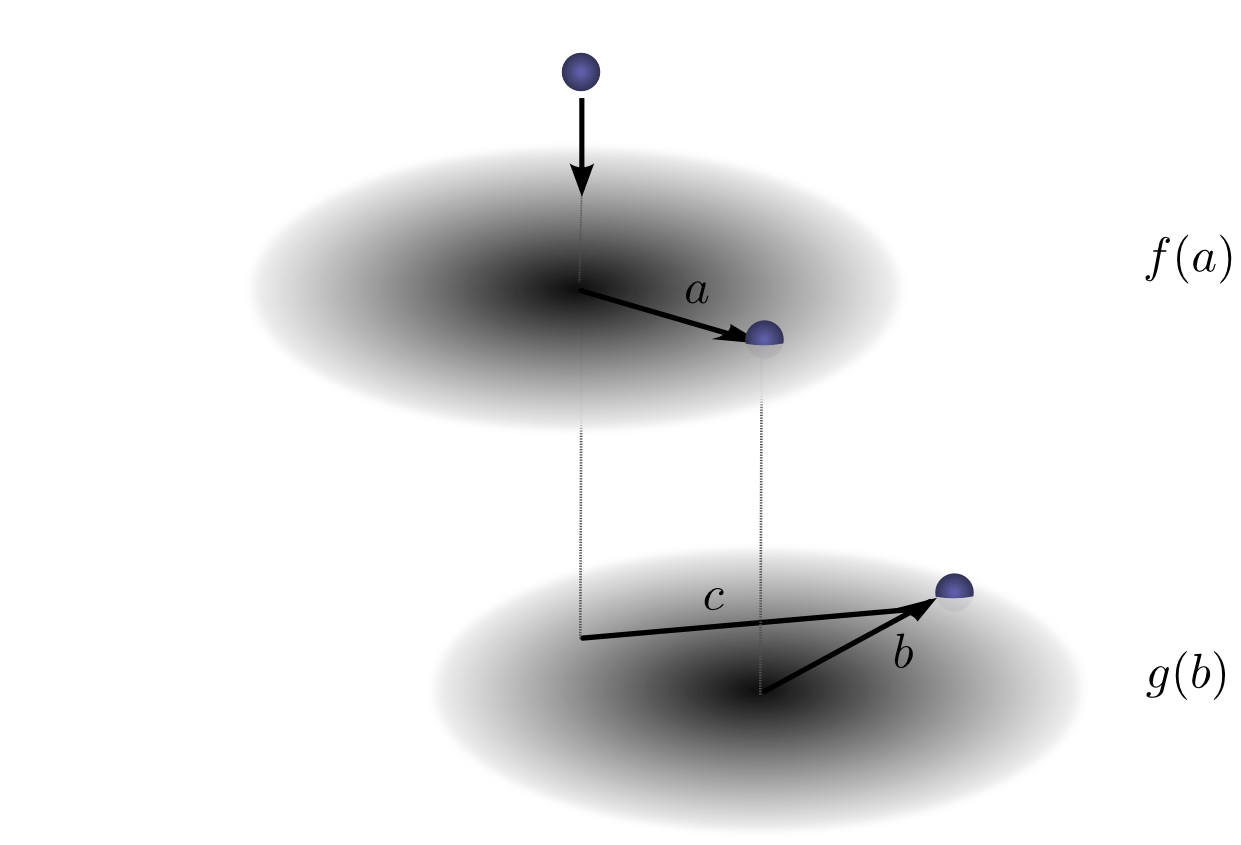Convolution is the same as before:

(fg)(c)=a+b=cf(a)g(b)(f∗g)(c)=∑a+b=cf(a)⋅g(b)

Except, now aa, bb and cc are vectors. To be more explicit,

(fg)(c1,c2)=a1+b1=c1a2+b2=c2f(a1,a2)g(b1,b2)(f∗g)(c1,c2)=∑a1+b1=c1a2+b2=c2f(a1,a2)⋅g(b1,b2)

Or in the standard definition:

(fg)(c1,c2)=a1,a2f(a1,a2)g(c1a1, c2a2)(f∗g)(c1,c2)=∑a1,a2f(a1,a2)⋅g(c1−a1, c2−a2)

Just like one-dimensional convolutions, we can think of a two-dimensional convolution as sliding one function on top of another, multiplying and adding.

One common application of this is image processing. We can think of images as two-dimensional functions. Many important image transformations are convolutions where you convolve the image function with a very small, local function called a “kernel.”The kernel slides to every position of the image and computes a new pixel as a weighted sum of the pixels it floats over.

For example, by averaging a 3×3 box of pixels, we can blur an image. To do this, our kernel takes the value 1/91/9 on each pixel in the box,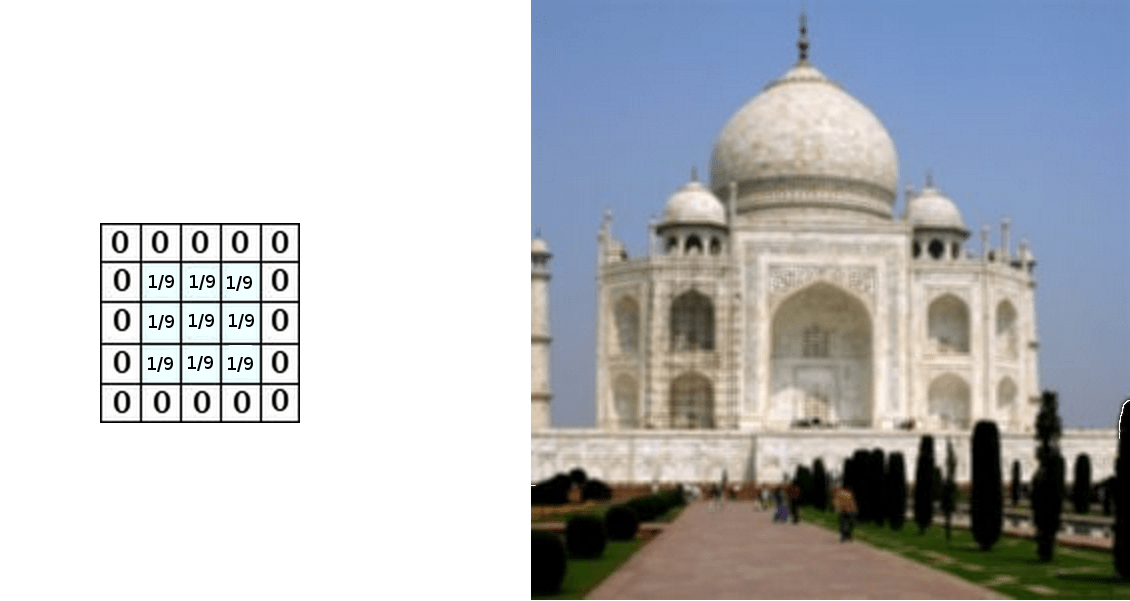Derived from the Gimp documentation

We can also detect edges by taking the values 1−1 and 11 on two adjacent pixels, and zero everywhere else. That is, we subtract two adjacent pixels. When side by side pixels are similar, this is gives us approximately zero. On edges, however, adjacent pixels are very different in the direction perpendicular to the edge.Derived from the Gimp documentation

The gimp documentation has many other examples.

## Convolutional Neural Networks

So, how does convolution relate to convolutional neural networks?

Consider a 1-dimensional convolutional layer with inputs {xn}{xn} and outputs {yn}{yn}, like we discussed in the previous post: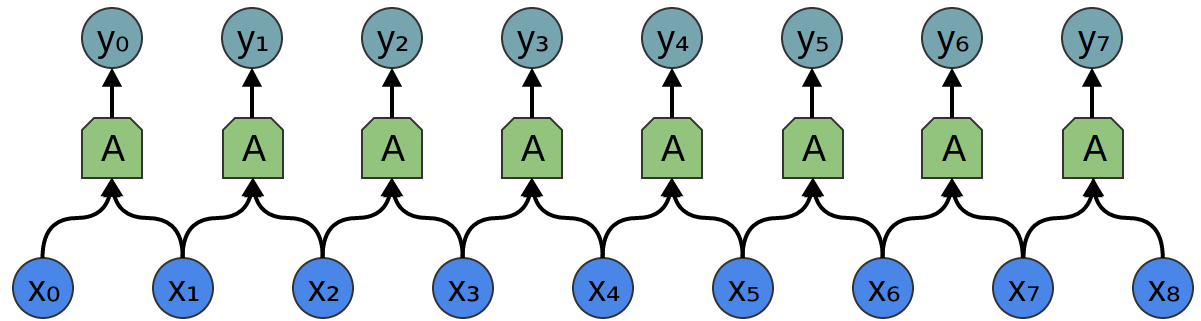As we observed, we can describe the outputs in terms of the inputs:

yn=A(xn,xn+1,...)yn=A(xn,xn+1,…)

Generally, AA would be multiple neurons. But suppose it is a single neuron for a moment.

Recall that a typical neuron in a neural network is described by:

σ(w0x0+w1x1+w2x2 ... +b)σ(w0x0+w1x1+w2x2 … +b)

Where x0x0, x1x1… are the inputs. The weights, w0w0, w1w1, … describe how the neuron connects to its inputs. A negative weight means that an input inhibits the neuron from firing, while a positive weight encourages it to. The weights are the heart of the neuron, controlling its behavior.3 Saying that multiple neurons are identical is the same thing as saying that the weights are the same.

It’s this wiring of neurons, describing all the weights and which ones are identical, that convolution will handle for us.

Typically, we describe all the neurons in a layer at once, rather than individually. The trick is to have a weight matrix, WW:

y=σ(Wx+b)y=σ(Wx+b)

For example, we get:

y0=σ(W0,0x0+W0,1x1+W0,2x2...)y0=σ(W0,0x0+W0,1×1+W0,2×2…)
y1=σ(W1,0x0+W1,1x1+W1,2x2...)y1=σ(W1,0x0+W1,1×1+W1,2×2…)

Each row of the matrix describes the weights connecting a neuron to its inputs.

Returning to the convolutional layer, though, because there are multiple copies of the same neuron, many weights appear in multiple positions.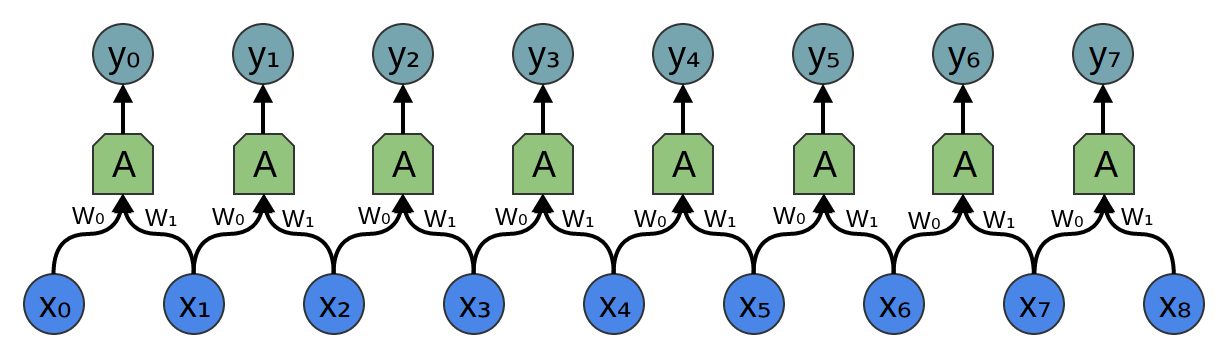Which corresponds to the equations:

y0=σ(W0x0+W1x1b)y0=σ(W0x0+W1x1−b)
y1=σ(W0x1+W1x2b)y1=σ(W0x1+W1x2−b)

So while, normally, a weight matrix connects every input to every neuron with different weights:

W=⎡⎣⎢⎢⎢⎢⎢⎢W0,0W1,0W2,0W3,0...W0,1W1,1W2,1W3,1...W0,2W1,2W2,2W3,2...W0,3W1,3W2,3W3,3..................
⎦⎥⎥⎥⎥⎥⎥W=

[W0,0W0,1W0,2W0,3…W1,0W1,1W1,2W1,3…W2,0W2,1W2,2W2,3…W3,0W3,1W3,2W3,3………………]

The matrix for a convolutional layer like the one above looks quite different. The same weights appear in a bunch of positions. And because neurons don’t connect to many possible inputs, there’s lots of zeros.

W=⎡⎣⎢⎢⎢⎢⎢w0000...w1w000...0w1w00...00w1w0..................⎤⎦⎥⎥⎥⎥⎥W=[w0w100…0w0w10…00w0w1…000w0………………]

Multiplying by the above matrix is the same thing as convolving with [...0,w1,w0,0…][…0,w1,w0,0…]. The function sliding to different positions corresponds to having neurons at those positions.The wiring of a two dimensional convolutional layer corresponds to a two-dimensional convolution.

Consider our example of using a convolution to detect edges in an image, above, by sliding a kernel around and applying it to every patch. Just like this, a convolutional layer will apply a neuron to every patch of the image.

## Conclusion

We introduced a lot of mathematical machinery in this blog post, but it may not be obvious what we gained. Convolution is obviously a useful tool in probability theory and computer graphics, but what do we gain from phrasing convolutional neural networks in terms of convolutions?

The first advantage is that we have some very powerful language for describing the wiring of networks. The examples we’ve dealt with so far haven’t been complicated enough for this benefit to become clear, but convolutions will allow us to get rid of huge amounts of unpleasant book-keeping for us.

Secondly, convolutions come with significant implementational advantages. Many libraries provide highly efficient convolution routines. Further, while convolution naively appears to be an O(n2)O(n2) operation, using some rather deep mathematical insights, it is possible to create a O(nlog(n))O(nlog⁡(n)) implementation. We will discuss this in much greater detail in a future post.

In fact, the use of highly-efficient parallel convolution implementations on GPUs has been essential to recent progress in computer vision.

## Next Posts in this Series

This post is part of a series on convolutional neural networks and their generalizations. The first two posts will be review for those familiar with deep learning, while later ones should be of interest to everyone. To get updates, subscribe to my RSS feed!

Please comment below or on the side. Pull requests can be made on github.

## Acknowledgments

I’m extremely grateful to Eliana Lorch, for extensive discussion of convolutions and help writing this post.

I’m also grateful to Michael Nielsen and Dario Amodei for their comments and support.

1. We want the probability of the ball rolling aa units the first time and also rolling bb units the second time. The distributions P(A)=f(a)P(A)=f(a) and P(b)=g(b)P(b)=g(b) are independent, with both distributions centered at 0. So P(a,b)=P(a)P(b)=f(a)g(b)P(a,b)=P(a)∗P(b)=f(a)⋅g(b).

2. The non-standard definition, which I haven’t previously seen, seems to have a lot of benefits. In future posts, we will find this definition very helpful because it lends itself to generalization to new algebraic structures. But it also has the advantage that it makes a lot of algebraic properties of convolutions really obvious.

For example, convolution is a commutative operation. That is, fg=gff∗g=g∗f. Why?

a+b=cf(a)g(b)  = b+a=cg(b)f(a)∑a+b=cf(a)⋅g(b)  = ∑b+a=cg(b)⋅f(a)

Convolution is also associative. That is, (fg)h=f(gh)(f∗g)∗h=f∗(g∗h). Why?

(a+b)+c=d(f(a)g(b))h(c)  = a+(b+c)=df(a)(g(b)h(c))∑(a+b)+c=d(f(a)⋅g(b))⋅h(c)  = ∑a+(b+c)=df(a)⋅(g(b)⋅h(c))

3. There’s also the bias, which is the “threshold” for whether the neuron fires, but it’s much simpler and I don’t want to clutter this section talking about it.

### An Intuitive Explanation of Convolutional Neural Networks

#### What are Convolutional Neural Networks and why are they important?

Convolutional Neural Networks (ConvNets or CNNs) are a category of Neural Networks that have proven very effective in areas such as image recognition and classification. ConvNets have been successful in identifying faces, objects and traffic signs apart from powering vision in robots and self driving cars.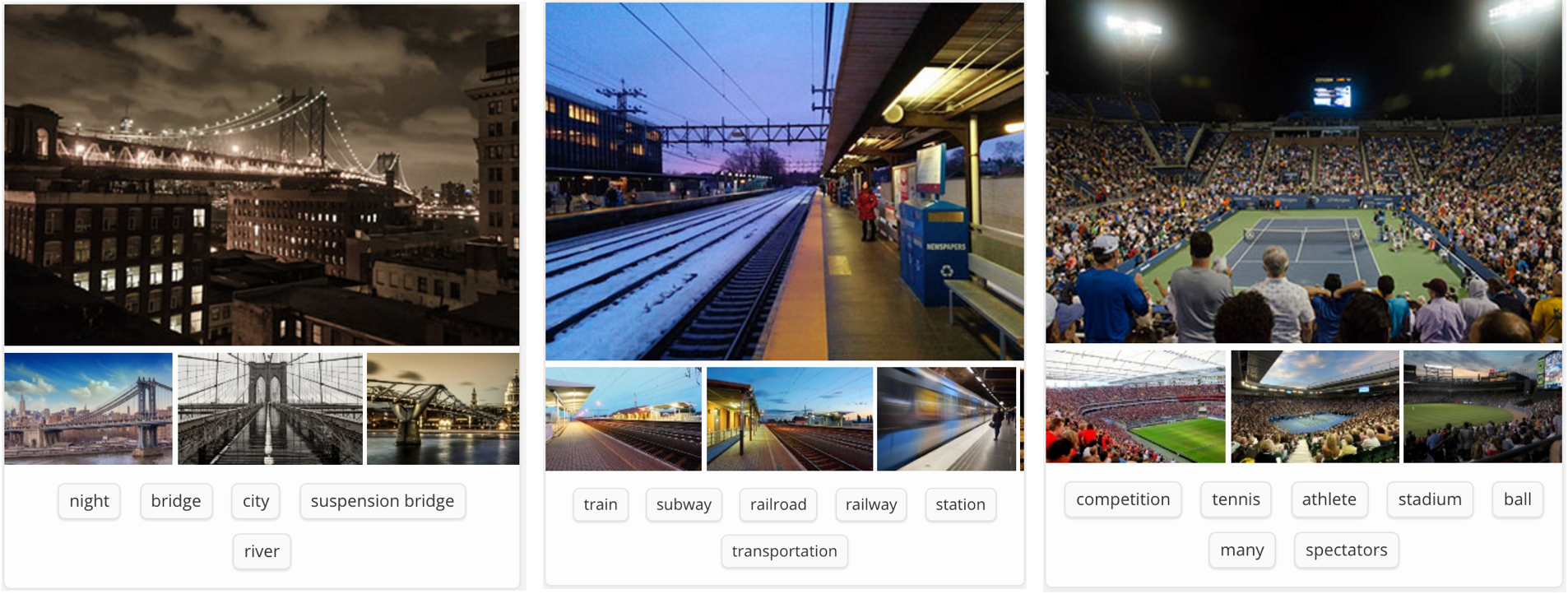###### Figure 1: Source 

In Figure 1 above, a ConvNet is able to recognize scenes and the system is able to suggest relevant tags such as ‘bridge’, ‘railway’ and ‘tennis’ while Figure 2 shows an example of ConvNets being used for recognizing everyday objects, humans and animals. Lately, ConvNets have been effective in several Natural Language Processing tasks (such as sentence classification) as well.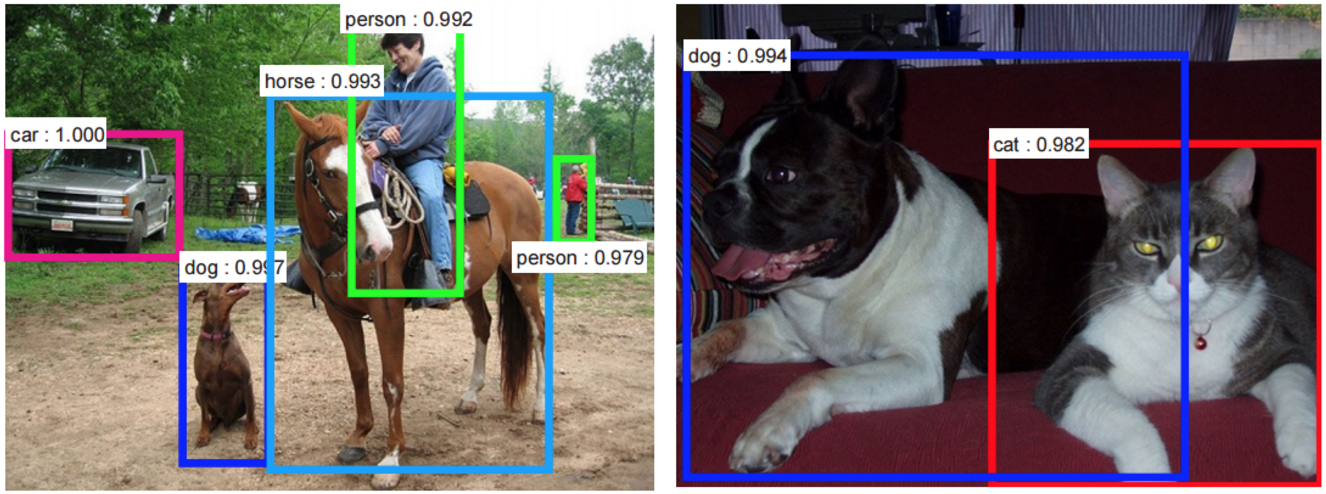###### Figure 2: Source 

ConvNets, therefore, are an important tool for most machine learning practitioners today. However, understanding ConvNets and learning to use them for the first time can sometimes be an intimidating experience. The primary purpose of this blog post is to develop an understanding of how Convolutional Neural Networks work on images.

If you are new to neural networks in general, I would recommend reading this short tutorial on Multi Layer Perceptrons to get an idea about how they work, before proceeding. Multi Layer Perceptrons are referred to as “Fully Connected Layers” in this post.

#### The LeNet Architecture (1990s)

LeNet was one of the very first convolutional neural networks which helped propel the field of Deep Learning. This pioneering work by Yann LeCun was named LeNet5 after many previous successful iterations since the year 1988 . At that time the LeNet architecture was used mainly for character recognition tasks such as reading zip codes, digits, etc.

Below, we will develop an intuition of how the LeNet architecture learns to recognize images. There have been several new architectures proposed in the recent years which are improvements over the LeNet, but they all use the main concepts from the LeNet and are relatively easier to understand if you have a clear understanding of the former.###### Figure 3: A simple ConvNet. Source 

The Convolutional Neural Network in Figure 3 is similar in architecture to the original LeNet and classifies an input image into four categories: dog, cat, boat or bird (the original LeNet was used mainly for character recognition tasks). As evident from the figure above, on receiving a boat image as input, the network correctly assigns the highest probability for boat (0.94) among all four categories. The sum of all probabilities in the output layer should be one (explained later in this post).

There are four main operations in the ConvNet shown in Figure 3 above:

1. Convolution
2. Non Linearity (ReLU)
3. Pooling or Sub Sampling
4. Classification (Fully Connected Layer)

These operations are the basic building blocks of every Convolutional Neural Network, so understanding how these work is an important step to developing a sound understanding of ConvNets. We will try to understand the intuition behind each of these operations below.

#### Images are a matrix of pixel values

Essentially, every image can be represented as a matrix of pixel values.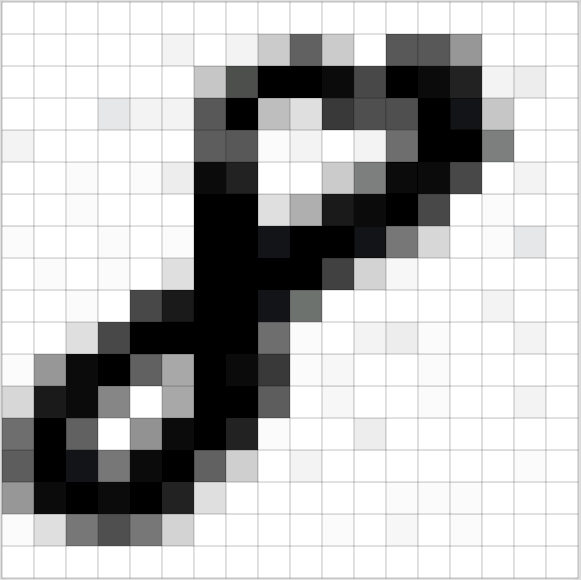###### Figure 4: Every image is a matrix of pixel values. Source 

Channel is a conventional term used to refer to a certain component of an image. An image from a standard digital camera will have three channels – red, green and blue – you can imagine those as three 2d-matrices stacked over each other (one for each color), each having pixel values in the range 0 to 255.

grayscale image, on the other hand, has just one channel. For the purpose of this post, we will only consider grayscale images, so we will have a single 2d matrix representing an image. The value of each pixel in the matrix will range from 0 to 255 – zero indicating black and 255 indicating white.

#### The Convolution Step

ConvNets derive their name from the “convolution” operator. The primary purpose of Convolution in case of a ConvNet is to extract features from the input image. Convolution preserves the spatial relationship between pixels by learning image features using small squares of input data. We will not go into the mathematical details of Convolution here, but will try to understand how it works over images.

As we discussed above, every image can be considered as a matrix of pixel values. Consider a 5 x 5 image whose pixel values are only 0 and 1 (note that for a grayscale image, pixel values range from 0 to 255, the green matrix below is a special case where pixel values are only 0 and 1):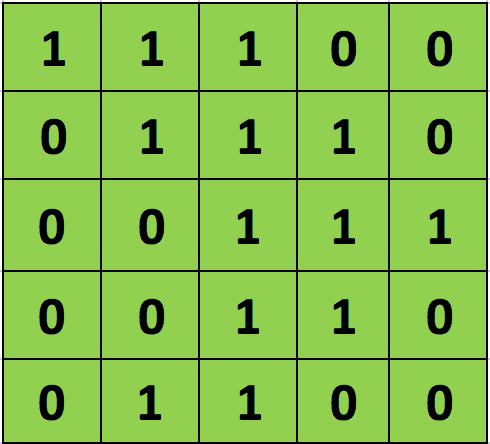Also, consider another 3 x 3 matrix as shown below: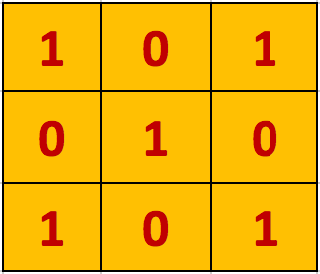Then, the Convolution of the 5 x 5 image and the 3 x 3 matrix can be computed as shown in the animation in Figure 5 below: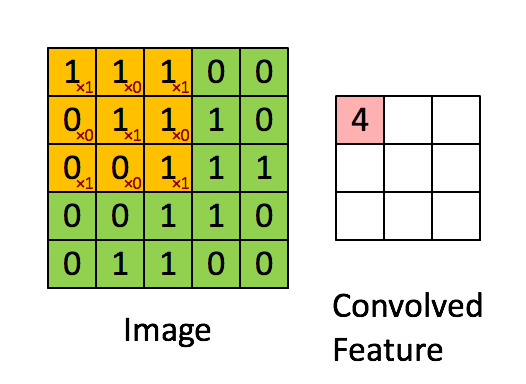###### Figure 5: The Convolution operation. The output matrix is called Convolved Feature or Feature Map. Source 

Take a moment to understand how the computation above is being done. We slide the orange matrix over our original image (green) by 1 pixel (also called ‘stride’) and for every position, we compute element wise multiplication (between the two matrices) and add the multiplication outputs to get the final integer which forms a single element of the output matrix (pink). Note that the 3×3 matrix “sees” only a part of the input image in each stride.

In CNN terminology, the 3×3 matrix is called a ‘filter‘ or ‘kernel’ or ‘feature detector’ and the matrix formed by sliding the filter over the image and computing the dot product is called the ‘Convolved Feature’ or ‘Activation Map’ or the ‘Feature Map‘. It is important to note that filters acts as feature detectors from the original input image.

It is evident from the animation above that different values of the filter matrix will produce different Feature Maps for the same input image. As an example, consider the following input image: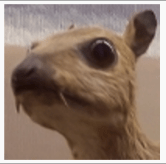In the table below, we can see the effects of convolution of the above image with different filters. As shown, we can perform operations such as Edge Detection, Sharpen and Blur just by changing the numeric values of our filter matrix before the convolution operation  – this means that different filters can detect different features from an image, for example edges, curves etc. More such examples are available in Section 8.2.4 here.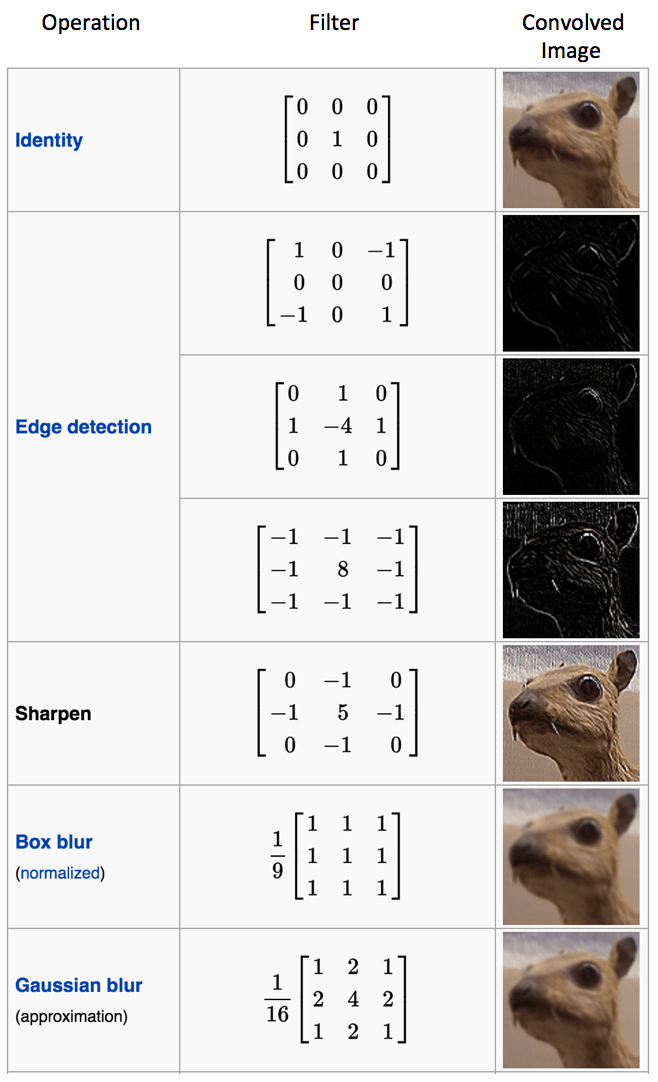Another good way to understand the Convolution operation is by looking at the animation in Figure 6 below:###### Figure 6: The Convolution Operation. Source 

A filter (with red outline) slides over the input image (convolution operation) to produce a feature map. The convolution of another filter (with the green outline), over the same image gives a different feature map as shown. It is important to note that the Convolution operation captures the local dependencies in the original image. Also notice how these two different filters generate different feature maps from the same original image. Remember that the image and the two filters above are just numeric matrices as we have discussed above.

In practice, a CNN learns the values of these filters on its own during the training process (although we still need to specify parameters such as number of filters, filter size, architecture of the network etc. before the training process). The more number of filters we have, the more image features get extracted and the better our network becomes at recognizing patterns in unseen images.

The size of the Feature Map (Convolved Feature) is controlled by three parameters  that we need to decide before the convolution step is performed:

• Depth: Depth corresponds to the number of filters we use for the convolution operation. In the network shown in Figure 7, we are performing convolution of the original boat image using three distinct filters, thus producing three different feature maps as shown. You can think of these three feature maps as stacked 2d matrices, so, the ‘depth’ of the feature map would be three.###### Figure 7
• Stride: Stride is the number of pixels by which we slide our filter matrix over the input matrix. When the stride is 1 then we move the filters one pixel at a time. When the stride is 2, then the filters jump 2 pixels at a time as we slide them around. Having a larger stride will produce smaller feature maps.
• Zero-padding: Sometimes, it is convenient to pad the input matrix with zeros around the border, so that we can apply the filter to bordering elements of our input image matrix. A nice feature of zero padding is that it allows us to control the size of the feature maps. Adding zero-padding is also called wide convolution, and not using zero-padding would be a narrow convolution. This has been explained clearly in .

#### Introducing Non Linearity (ReLU)

An additional operation called ReLU has been used after every Convolution operation in Figure 3 above. ReLU stands for Rectified Linear Unit and is a non-linear operation. Its output is given by: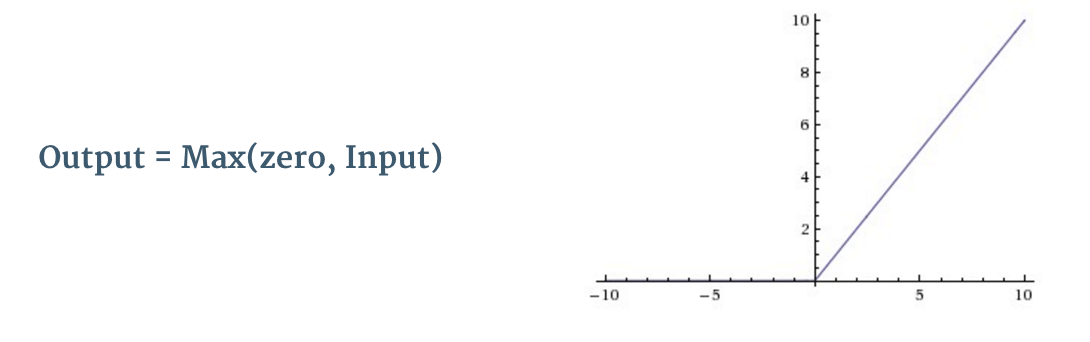###### Figure 8: the ReLU operation

ReLU is an element wise operation (applied per pixel) and replaces all negative pixel values in the feature map by zero. The purpose of ReLU is to introduce non-linearity in our ConvNet, since most of the real-world data we would want our ConvNet to learn would be non-linear (Convolution is a linear operation – element wise matrix multiplication and addition, so we account for non-linearity by introducing a non-linear function like ReLU).

The ReLU operation can be understood clearly from Figure 9 below. It shows the ReLU operation applied to one of the feature maps obtained in Figure 6 above. The output feature map here is also referred to as the ‘Rectified’ feature map.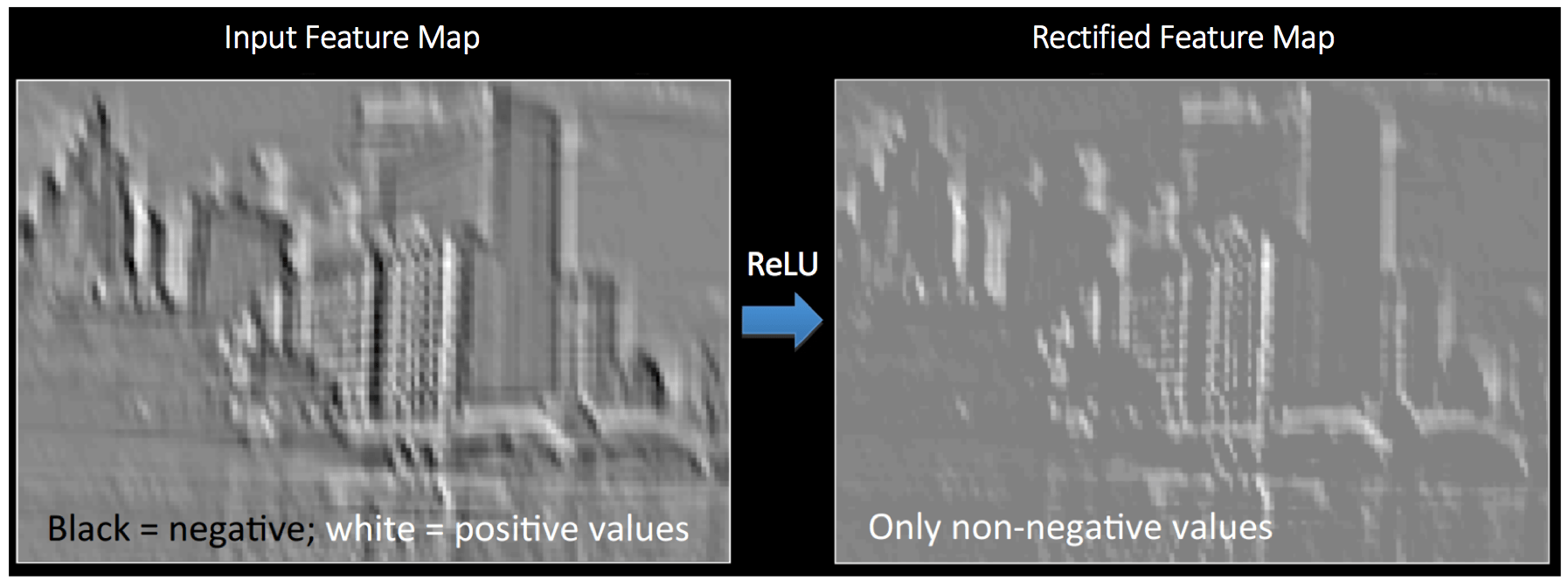###### Figure 9: ReLU operation. Source 

Other non linear functions such as tanh or sigmoid can also be used instead of ReLU, but ReLU has been found to perform better in most situations.

#### The Pooling Step

Spatial Pooling (also called subsampling or downsampling) reduces the dimensionality of each feature map but retains the most important information. Spatial Pooling can be of different types: Max, Average, Sum etc.

In case of Max Pooling, we define a spatial neighborhood (for example, a 2×2 window) and take the largest element from the rectified feature map within that window. Instead of taking the largest element we could also take the average (Average Pooling) or sum of all elements in that window. In practice, Max Pooling has been shown to work better.

Figure 10 shows an example of Max Pooling operation on a Rectified Feature map (obtained after convolution + ReLU operation) by using a 2×2 window.###### Figure 10: Max Pooling. Source 

We slide our 2 x 2 window by 2 cells (also called ‘stride’) and take the maximum value in each region. As shown in Figure 10, this reduces the dimensionality of our feature map.

In the network shown in Figure 11, pooling operation is applied separately to each feature map (notice that, due to this, we get three output maps from three input maps).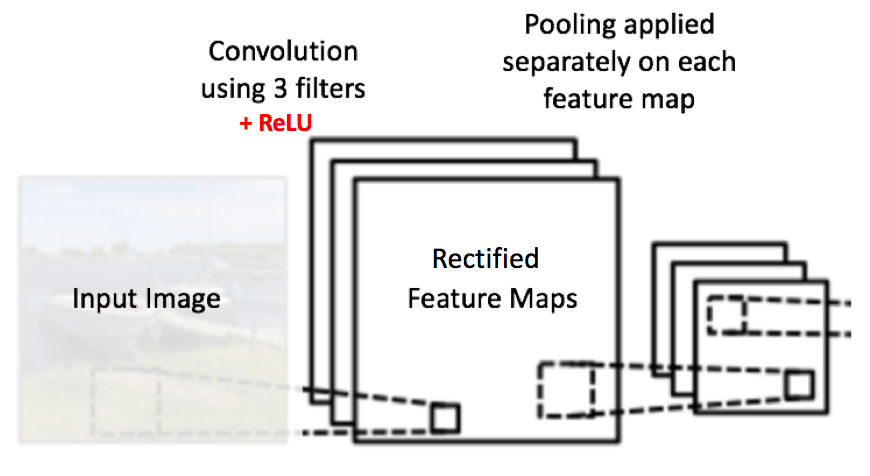###### Figure 11: Pooling applied to Rectified Feature Maps

Figure 12 shows the effect of Pooling on the Rectified Feature Map we received after the ReLU operation in Figure 9 above.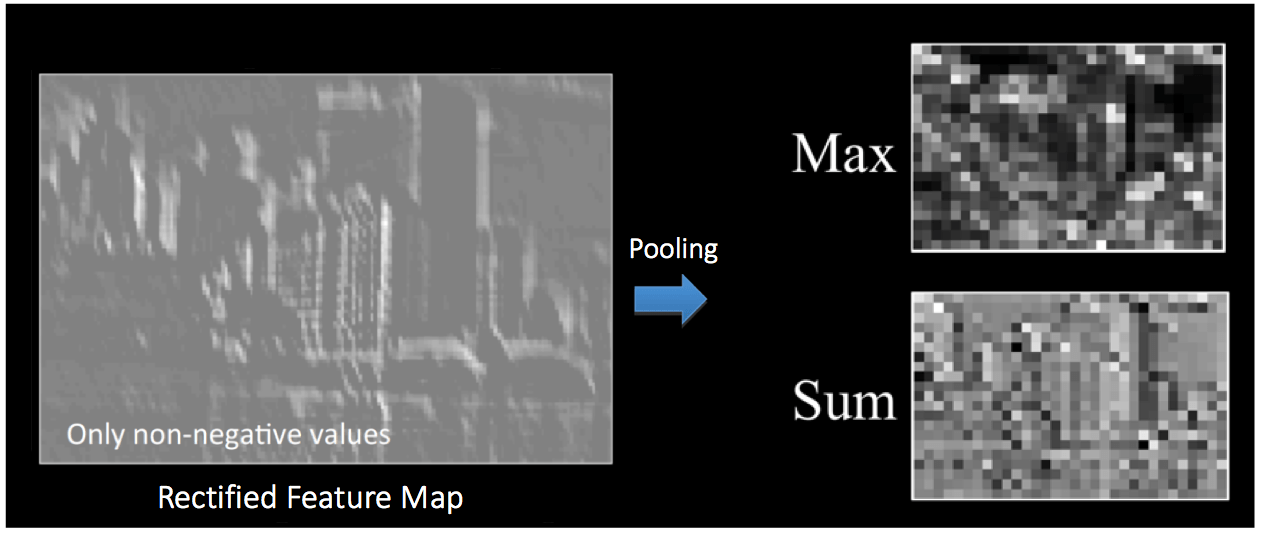###### Figure 12: Pooling. Source 

The function of Pooling is to progressively reduce the spatial size of the input representation . In particular, pooling

• makes the input representations (feature dimension) smaller and more manageable
• reduces the number of parameters and computations in the network, therefore, controlling overfitting 
• makes the network invariant to small transformations, distortions and translations in the input image (a small distortion in input will not change the output of Pooling – since we take the maximum / average value in a local neighborhood).
• helps us arrive at an almost scale invariant representation of our image (the exact term is “equivariant”). This is very powerful since we can detect objects in an image no matter where they are located (read  and  for details).

#### Story so far###### Figure 13

So far we have seen how Convolution, ReLU and Pooling work. It is important to understand that these layers are the basic building blocks of any CNN. As shown in Figure 13, we have two sets of Convolution, ReLU & Pooling layers – the 2nd Convolution layer performs convolution on the output of the first Pooling Layer using six filters to produce a total of six feature maps. ReLU is then applied individually on all of these six feature maps. We then perform Max Pooling operation separately on each of the six rectified feature maps.

Together these layers extract the useful features from the images, introduce non-linearity in our network and reduce feature dimension while aiming to make the features somewhat equivariant to scale and translation .

The output of the 2nd Pooling Layer acts as an input to the Fully Connected Layer, which we will discuss in the next section.

#### Fully Connected Layer

The Fully Connected layer is a traditional Multi Layer Perceptron that uses a softmax activation function in the output layer (other classifiers like SVM can also be used, but will stick to softmax in this post). The term “Fully Connected” implies that every neuron in the previous layer is connected to every neuron on the next layer. I recommend reading this post if you are unfamiliar with Multi Layer Perceptrons.

The output from the convolutional and pooling layers represent high-level features of the input image. The purpose of the Fully Connected layer is to use these features for classifying the input image into various classes based on the training dataset. For example, the image classification task we set out to perform has four possible outputs as shown in Figure 14 below (note that Figure 14 does not show connections between the nodes in the fully connected layer)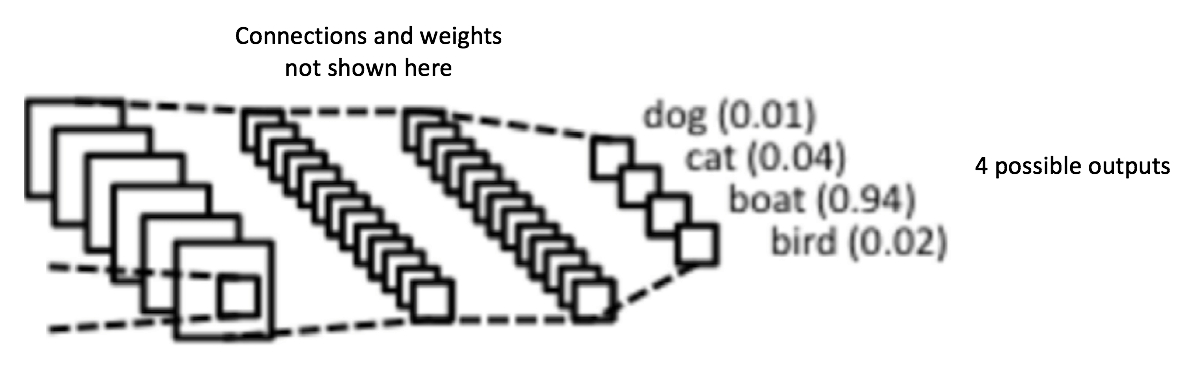###### Figure 14: Fully Connected Layer -each node is connected to every other node in the adjacent layer

Apart from classification, adding a fully-connected layer is also a (usually) cheap way of learning non-linear combinations of these features. Most of the features from convolutional and pooling layers may be good for the classification task, but combinations of those features might be even better .

The sum of output probabilities from the Fully Connected Layer is 1. This is ensured by using the Softmax as the activation function in the output layer of the Fully Connected Layer. The Softmax function takes a vector of arbitrary real-valued scores and squashes it to a vector of values between zero and one that sum to one.

#### Putting it all together – Training using Backpropagation

As discussed above, the Convolution + Pooling layers act as Feature Extractors from the input image while Fully Connected layer acts as a classifier.

Note that in Figure 15 below, since the input image is a boat, the target probability is 1 for Boat class and 0 for other three classes, i.e.

• Input Image = Boat
• Target Vector = [0, 0, 1, 0]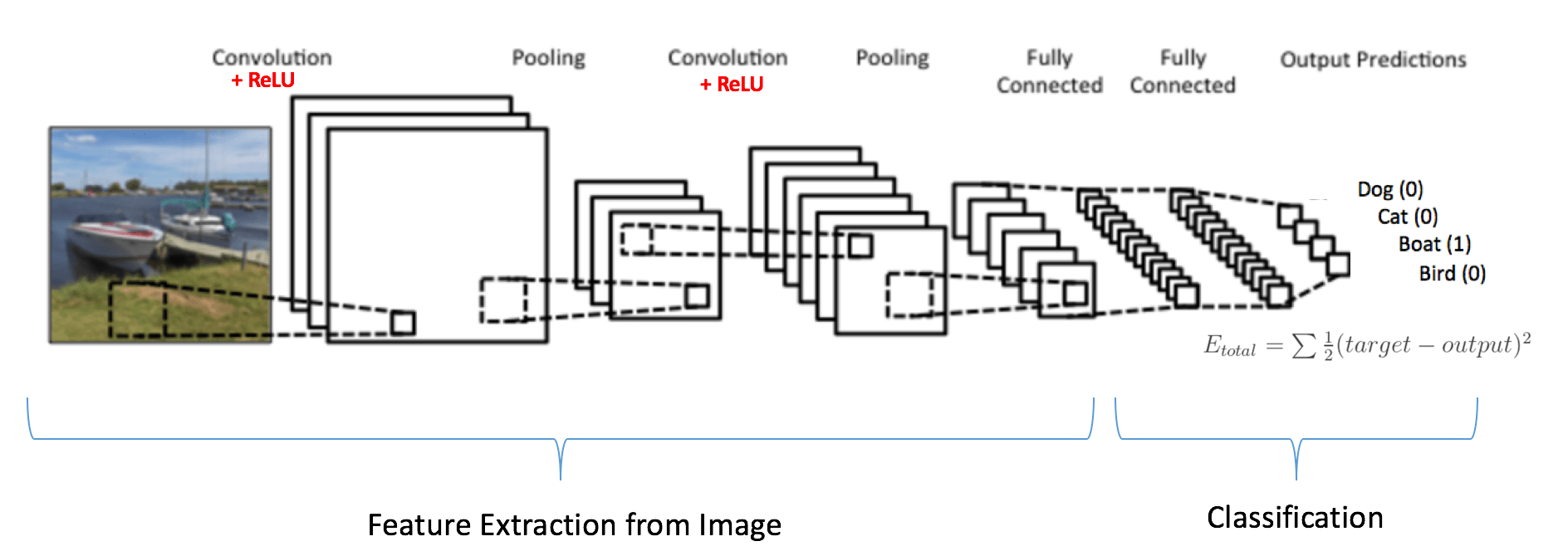###### Figure 15: Training the ConvNet

The overall training process of the Convolution Network may be summarized as below:

• Step1: We initialize all filters and parameters / weights with random values
• Step2: The network takes a training image as input, goes through the forward propagation step (convolution, ReLU and pooling operations along with forward propagation in the Fully Connected layer) and finds the output probabilities for each class.
• Lets say the output probabilities for the boat image above are [0.2, 0.4, 0.1, 0.3]
• Since weights are randomly assigned for the first training example, output probabilities are also random.
• Step3: Calculate the total error at the output layer (summation over all 4 classes)
•  Total Error = ∑  ½ (target probability – output probability) ²
• Step4: Use Backpropagation to calculate the gradients of the error with respect to all weights in the network and use gradient descent to update all filter values / weights and parameter values to minimize the output error.
• The weights are adjusted in proportion to their contribution to the total error.
• When the same image is input again, output probabilities might now be [0.1, 0.1, 0.7, 0.1], which is closer to the target vector [0, 0, 1, 0].
• This means that the network has learnt to classify this particular image correctly by adjusting its weights / filters such that the output error is reduced.
• Parameters like number of filters, filter sizes, architecture of the network etc. have all been fixed before Step 1 and do not change during training process – only the values of the filter matrix and connection weights get updated.
• Step5: Repeat steps 2-4 with all images in the training set.

The above steps train the ConvNet – this essentially means that all the weights and parameters of the ConvNet have now been optimized to correctly classify images from the training set.

When a new (unseen) image is input into the ConvNet, the network would go through the forward propagation step and output a probability for each class (for a new image, the output probabilities are calculated using the weights which have been optimized to correctly classify all the previous training examples). If our training set is large enough, the network will (hopefully) generalize well to new images and classify them into correct categories.

Note 1: The steps above have been oversimplified and mathematical details have been avoided to provide intuition into the training process. See  and  for a mathematical formulation and thorough understanding.

Note 2: In the example above we used two sets of alternating Convolution and Pooling layers. Please note however, that these operations can be repeated any number of times in a single ConvNet. In fact, some of the best performing ConvNets today have tens of Convolution and Pooling layers! Also, it is not necessary to have a Pooling layer after every Convolutional Layer. As can be seen in the Figure 16 below, we can have multiple Convolution + ReLU operations in succession before having a Pooling operation. Also notice how each layer of the ConvNet is visualized in the Figure 16 below.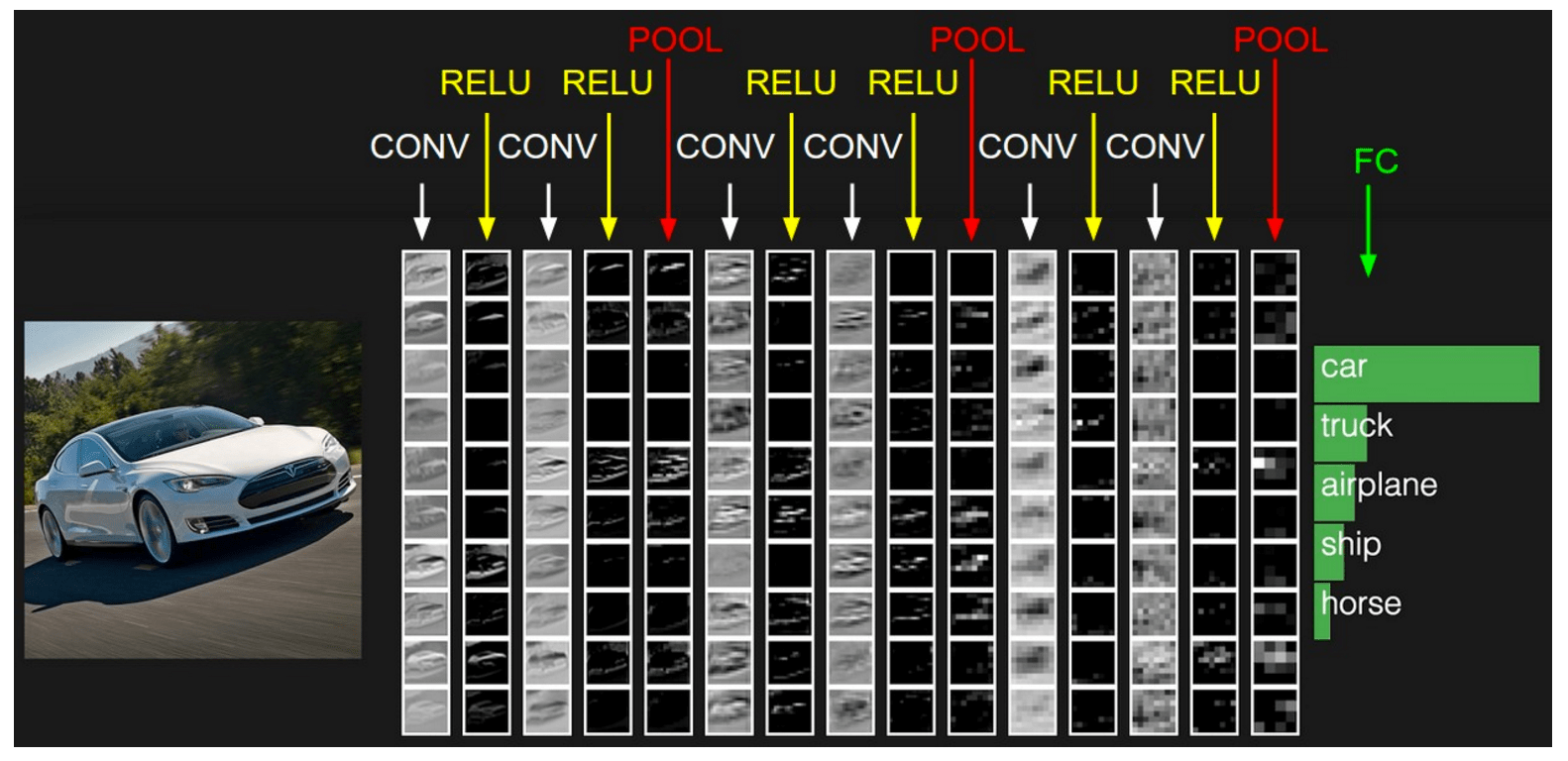#### Visualizing Convolutional Neural Networks

In general, the more convolution steps we have, the more complicated features our network will be able to learn to recognize. For example, in Image Classification a ConvNet may learn to detect edges from raw pixels in the first layer, then use the edges to detect simple shapes in the second layer, and then use these shapes to deter higher-level features, such as facial shapes in higher layers . This is demonstrated in Figure 17 below – these features were learnt using a Convolutional Deep Belief Network and the figure is included here just for demonstrating the idea (this is only an example: real life convolution filters may detect objects that have no meaning to humans).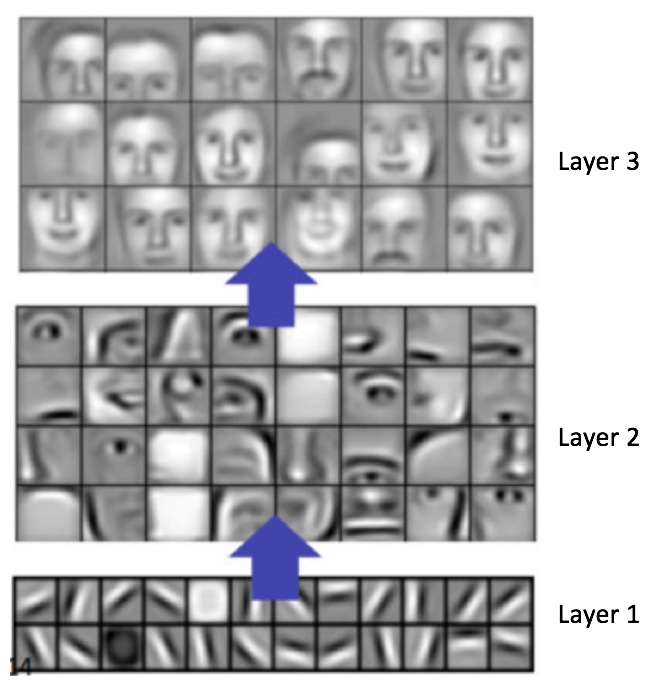###### Figure 17: Learned features from a Convolutional Deep Belief Network

Adam Harley created amazing visualizations of a Convolutional Neural Network trained on the MNIST Database of handwritten digits . I highly recommend playing around with it to understand details of how a CNN works.

We will see below how the network works for an input ‘8’. Note that the visualization in Figure 18 does not show the ReLU operation separately.###### Figure 18: Visualizing a ConvNet trained on handwritten digits

The input image contains 1024 pixels (32 x 32 image) and the first Convolution layer (Convolution Layer 1) is formed by convolution of six unique 5 × 5 (stride 1) filters with the input image. As seen, using six different filters produces a feature map of depth six.

Convolutional Layer 1 is followed by Pooling Layer 1 that does 2 × 2 max pooling (with stride 2) separately over the six feature maps in Convolution Layer 1. You can move your mouse pointer over any pixel in the Pooling Layer and observe the 4 x 4 grid it forms in the previous Convolution Layer (demonstrated in Figure 19). You’ll notice that the pixel having the maximum value (the brightest one) in the 4 x 4 grid makes it to the Pooling layer.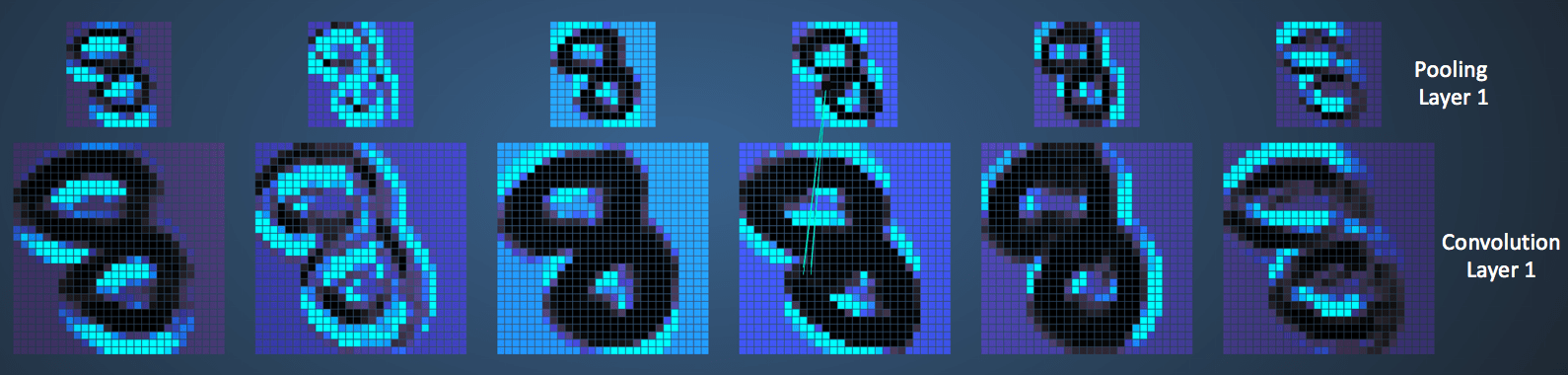###### Figure 19: Visualizing the Pooling Operation

Pooling Layer 1 is followed by sixteen 5 × 5 (stride 1) convolutional filters that perform the convolution operation. This is followed by Pooling Layer 2 that does 2 × 2 max pooling (with stride 2). These two layers use the same concepts as described above.

We then have three fully-connected (FC) layers. There are:

• 120 neurons in the first FC layer
• 100 neurons in the second FC layer
• 10 neurons in the third FC layer corresponding to the 10 digits – also called the Output layer

Notice how in Figure 20, each of the 10 nodes in the output layer are connected to all 100 nodes in the 2nd Fully Connected layer (hence the name Fully Connected).

Also, note how the only bright node in the Output Layer corresponds to ‘8’ – this means that the network correctly classifies our handwritten digit (brighter node denotes that the output from it is higher, i.e. 8 has the highest probability among all other digits).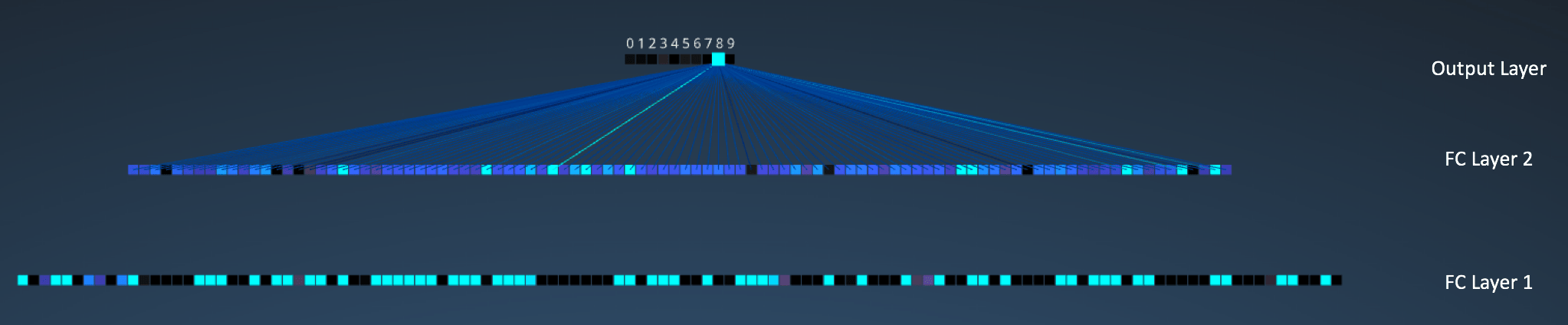###### Figure 20: Visualizing the Filly Connected Layers

The 3d version of the same visualization is available here.

#### Other ConvNet Architectures

Convolutional Neural Networks have been around since early 1990s. We discussed the LeNet above which was one of the very first convolutional neural networks. Some other influential architectures are listed below  .

• 1990s to 2012: In the years from late 1990s to early 2010s convolutional neural network were in incubation. As more and more data and computing power became available, tasks that convolutional neural networks could tackle became more and more interesting.
• AlexNet (2012) – In 2012, Alex Krizhevsky (and others) released AlexNet which was a deeper and much wider version of the LeNet and won by a large margin the difficult ImageNet Large Scale Visual Recognition Challenge (ILSVRC) in 2012. It was a significant breakthrough with respect to the previous approaches and the current widespread application of CNNs can be attributed to this work.
• ZF Net (2013) – The ILSVRC 2013 winner was a Convolutional Network from Matthew Zeiler and Rob Fergus. It became known as the ZFNet (short for Zeiler & Fergus Net). It was an improvement on AlexNet by tweaking the architecture hyperparameters.
• GoogLeNet (2014) – The ILSVRC 2014 winner was a Convolutional Network from Szegedy et al. from Google. Its main contribution was the development of an Inception Module that dramatically reduced the number of parameters in the network (4M, compared to AlexNet with 60M).
• VGGNet (2014) – The runner-up in ILSVRC 2014 was the network that became known as the VGGNet. Its main contribution was in showing that the depth of the network (number of layers) is a critical component for good performance.
• ResNets (2015) – Residual Network developed by Kaiming He (and others) was the winner of ILSVRC 2015. ResNets are currently by far state of the art Convolutional Neural Network models and are the default choice for using ConvNets in practice (as of May 2016).
• DenseNet (August 2016) – Recently published by Gao Huang (and others), the Densely Connected Convolutional Network has each layer directly connected to every other layer in a feed-forward fashion. The DenseNet has been shown to obtain significant improvements over previous state-of-the-art architectures on five highly competitive object recognition benchmark tasks. Check out the Torch implementation here.

#### Conclusion

In this post, I have tried to explain the main concepts behind Convolutional Neural Networks in simple terms. There are several details I have oversimplified / skipped, but hopefully this post gave you some intuition around how they work.

This post was originally inspired from Understanding Convolutional Neural Networks for NLP by Denny Britz (which I would recommend reading) and a number of explanations here are based on that post. For a more thorough understanding of some of these concepts, I would encourage you to go through the notes from Stanford’s course on ConvNets as well as other excellent resources mentioned under References below. If you face any issues understanding any of the above concepts or have questions / suggestions, feel free to leave a comment below.

All images and animations used in this post belong to their respective authors as listed in References section below.

#### References

2. Shaoqing Ren, et al, “Faster R-CNN: Towards Real-Time Object Detection with Region Proposal Networks”, 2015, arXiv:1506.01497
3. Neural Network Architectures, Eugenio Culurciello’s blog
4. CS231n Convolutional Neural Networks for Visual Recognition, Stanford
5. Clarifai / Technology
6. Machine Learning is Fun! Part 3: Deep Learning and Convolutional Neural Networks
7. Feature extraction using convolution, Stanford
8. Wikipedia article on Kernel (image processing)
9. Deep Learning Methods for Vision, CVPR 2012 Tutorial
10. Neural Networks by Rob Fergus, Machine Learning Summer School 2015
11. What do the fully connected layers do in CNNs?
12. Convolutional Neural Networks, Andrew Gibiansky
13. A. W. Harley, “An Interactive Node-Link Visualization of Convolutional Neural Networks,” in ISVC, pages 867-877, 2015 (link)
14. Understanding Convolutional Neural Networks for NLP
15. Backpropagation in Convolutional Neural Networks
16. A Beginner’s Guide To Understanding Convolutional Neural Networks
17. Vincent Dumoulin, et al, “A guide to convolution arithmetic for deep learning”, 2015, arXiv:1603.07285
18. What is the difference between deep learning and usual machine learning?
19. How is a convolutional neural network able to learn invariant features?
20. A Taxonomy of Deep Convolutional Neural Nets for Computer Vision